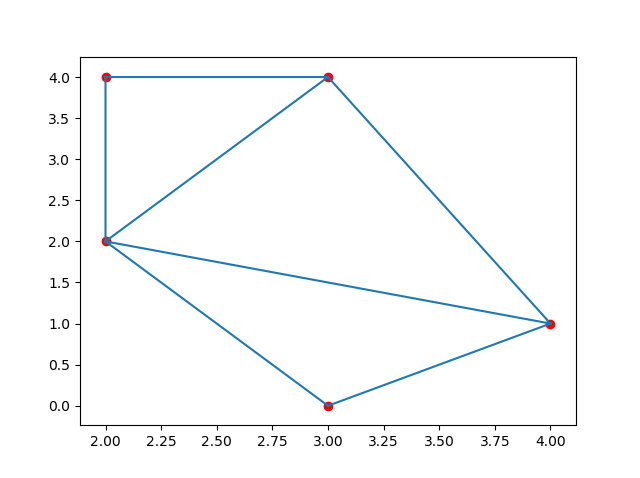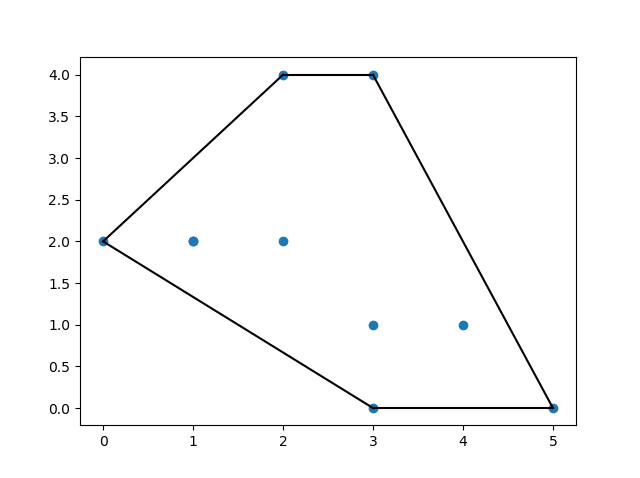# SciPy Spatial Data

## Working with Spatial Data

Spatial data refers to data that is represented in a geometric space.

E.g. points on a coordinate system.

We deal with spatial data problems on many tasks.

E.g. finding if a point is inside a boundary or not.

SciPy provides us with the module `scipy.spatial`, which has functions for working with spatial data.

## Triangulation

A Triangulation of a polygon is to divide the polygon into multiple triangles with which we can compute an area of the polygon.

A Triangulation with points means creating surface composed triangles in which all of the given points are on at least one vertex of any triangle in the surface.

One method to generate these triangulations through points is the `Delaunay()` Triangulation.

### Example

Create a triangulation from following points:

import numpy as np
from scipy.spatial import Delaunay
import matplotlib.pyplot as plt

points = np.array([
[2, 4],
[3, 4],
[3, 0],
[2, 2],
[4, 1]
])

simplices = Delaunay(points).simplices

plt.triplot(points[:, 0], points[:, 1], simplices)
plt.scatter(points[:, 0], points[:, 1], color='r')

plt.show()

### Result:Try it Yourself »

Note: The `simplices` property creates a generalization of the triangle notation.

## Convex Hull

A convex hull is the smallest polygon that covers all of the given points.

Use the `ConvexHull()` method to create a Convex Hull.

### Example

Create a convex hull for following points:

import numpy as np
from scipy.spatial import ConvexHull
import matplotlib.pyplot as plt

points = np.array([
[2, 4],
[3, 4],
[3, 0],
[2, 2],
[4, 1],
[1, 2],
[5, 0],
[3, 1],
[1, 2],
[0, 2]
])

hull = ConvexHull(points)
hull_points = hull.simplices

plt.scatter(points[:,0], points[:,1])
for simplex in hull_points:
plt.plot(points[simplex,0], points[simplex,1], 'k-')

plt.show()

### Result:Try it Yourself »

## KDTrees

KDTrees are a datastructure optimized for nearest neighbor queries.

E.g. in a set of points using KDTrees we can efficiently ask which points are nearest to a certain given point.

The `KDTree()` method returns a KDTree object.

The `query()` method returns the distance to the nearest neighbor and the location of the neighbors.

### Example

Find the nearest neighbor to point (1,1):

from scipy.spatial import KDTree

points = [(1, -1), (2, 3), (-2, 3), (2, -3)]

kdtree = KDTree(points)

res = kdtree.query((1, 1))

print(res)

### Result:

```
(2.0, 0)

```

Try it Yourself »

## Distance Matrix

There are many Distance Metrics used to find various types of distances between two points in data science, Euclidean distsance, cosine distsance etc.

The distance between two vectors may not only be the length of straight line between them, it can also be the angle between them from origin, or number of unit steps required etc.

Many of the Machine Learning algorithm's performance depends greatly on distance metrices. E.g. "K Nearest Neighbors", or "K Means" etc.

Let us look at some of the Distance Metrices:

## Euclidean Distance

Find the euclidean distance between given points.

### Example

from scipy.spatial.distance import euclidean

p1 = (1, 0)
p2 = (10, 2)

res = euclidean(p1, p2)

print(res)

### Result:

```
9.21954445729

```

Try it Yourself »

## Cityblock Distance (Manhattan Distance)

Is the distance computed using 4 degrees of movement.

E.g. we can only move: up, down, right, or left, not diagonally.

### Example

Find the cityblock distance between given points:

from scipy.spatial.distance import cityblock

p1 = (1, 0)
p2 = (10, 2)

res = cityblock(p1, p2)

print(res)

### Result:

```
11

```

Try it Yourself »

## Cosine Distance

Is the value of cosine angle between the two points A and B.

### Example

Find the cosine distsance between given points:

from scipy.spatial.distance import cosine

p1 = (1, 0)
p2 = (10, 2)

res = cosine(p1, p2)

print(res)

### Result:

```
0.019419324309079777

```

Try it Yourself »

## Hamming Distance

Is the proportion of bits where two bits are difference.

It's a way to measure distance for binary sequences.

### Example

Find the hamming distance between given points:

from scipy.spatial.distance import hamming

p1 = (True, False, True)
p2 = (False, True, True)

res = hamming(p1, p2)

print(res)

### Result:

```
0.666666666667

```

Try it Yourself »

## Exercise:

Consider this image:Which method is most likely to be used to find the smallest polygon that covers all of the given points?

```The () method
```

Start the Exercise